Next: Jacobi integral Up: Three-body problem Previous: Introduction

# Circular restricted three-body problem

Consider an isolated dynamical system consisting of three gravitationally interacting point masses,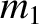,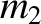, and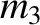. Suppose, however, that the third mass,, is so much smaller than the other two that it has a negligible effect on their motion. Suppose, further, that the first two masses,and, execute circular orbits about their common center of mass. In the following, we shall examine this simplified problem, which is usually referred to as the circular restricted three-body problem. The problem under investigation has obvious applications to the solar system. For instance, the first two masses might represent the Sun and a planet (recall that a given planet and the Sun do indeed execute almost circular orbits about their common center of mass), whereas the third mass might represent an asteroid or a comet (asteroids and comets do indeed have much smaller masses than the Sun or any of the planets).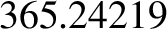Let us define a Cartesian coordinate system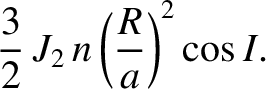in an inertial reference frame whose origin coincides with the center of mass,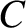, of the two orbiting masses,and. Furthermore, let the orbital plane of these masses coincide with the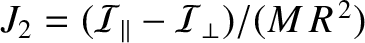-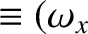plane, and let them both lie on the-axis at time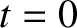. (See Figure 9.1.) Suppose thatis the constant distance between the two orbiting masses,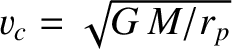the constant distance between massand the origin, and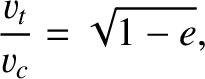the constant distance between massand the origin. Moreover, let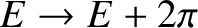be the constant orbital angular velocity. It follows, from Section 4.16, that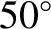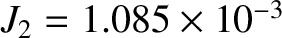(9.1) and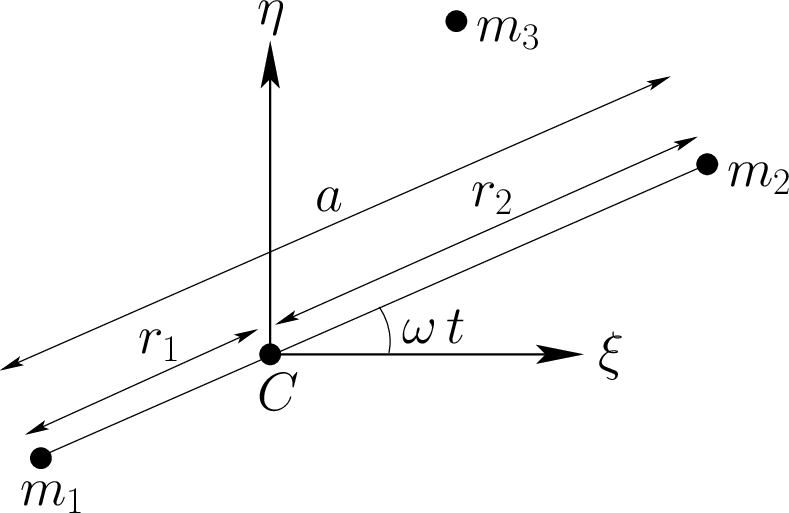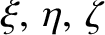(9.2)

where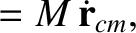.

It is convenient to choose our unit of length such that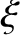, and our unit of mass such that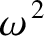. It follows, from Equation (9.1), that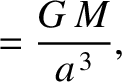. However, we shall continue to retainin our equations, for the sake of clarity. Let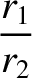and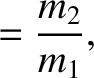. It is easily demonstrated that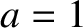and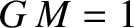. Hence, the two orbiting masses,and, have position vectors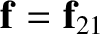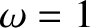(9.3) and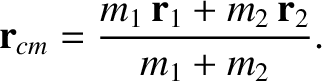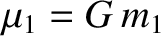(9.4)

respectively. (See Figure 9.1.) Let the third mass have position vector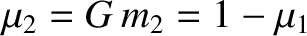. The Cartesian components of the equation of motion of this mass are thus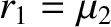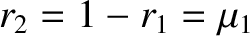(9.5)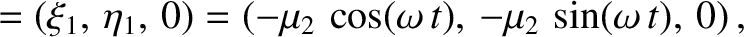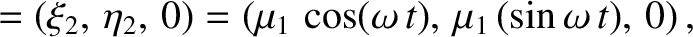(9.6) and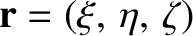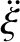(9.7)

where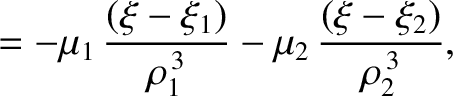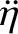(9.8) and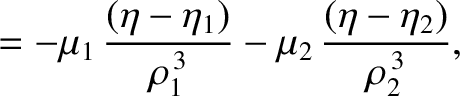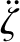(9.9)Next: Jacobi integral Up: Three-body problem Previous: Introduction
Richard Fitzpatrick 2016-03-31